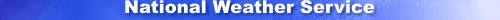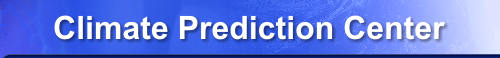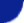efficiently. Climate Prediction Center - wgrib2: -set_scalingSite Map News Organization SearchHOME > Monitoring_and_Data > Oceanic and Atmospheric Data > Reanalysis: Atmospheric Data > wgrib2 -set_scaling

Introduction

The values at the grid points points are usually stored in in this format,

Y = (R + i*2**B)*(10**D)

R = reference value (32-bit IEEE floating point number)
i = integer, 0..2**N-1
N = binary bit precision
B = binary scaling, -127..127
D = decimal scaling, -127..127
There are 3 sytems for storing the number which I call

ECMWF convention: D = 0, N = parameter

Y = R + i*2**B
R = reference value
i = integer, 0..2**N-1
N = binary bit precision, a parameter
B = binary scaling, determined by grib routines

NCEP convention: B = parameter, D = parameter

R = reference value
i = integer, 0..2**N-1
N = binary bit precision, determined by grib routines
B = binary scaling, a parameter
D = decimal scaling, a parameter

Note, global model uses a variant: B = 0, D = parameter

Both the ECMWF and NCEP conventions have their advantages and disadvantages. The ECMWF method is easier to use, you just set the binary precision to N bits (12? 16?) for all variables and you are done. With the NCEP convention, you have to set the scaling for each variable separately. For some variables such as specific humidity, the scaling should be pressure dependent. On the other hand, if you are trying to get the smallest files, the NCEP convention is better. For example, you want to get the RH to the nearest integer. With the NCEP method, you simply set D = B = 0. For general use, I suggest that you use the ECMWF convention because people time is usually more valuable than disk space. Ok, I value my time more than a few GB. On the othe hand, I've been involved with more than my share of projects were disk space has been the critical issue.

By default, wgrib2 will encode using the ECMWF convention using 12 bits. The number of bits can be changed by the -set_bin_prec option. The -set_grib_max_bits option will have to be used if the binary precision is set to more than 16.

Default Scaling

When you read a field, the scaling of the field (B, D) are saved as the scaling parameter. However, Some options such as the -rpn option can change the magnitude of the field and scaling from the input field may not longer be appropriate. So these options will revert to the default scaling (ECMWF-style using the N bits). However, in some cases such as time-interpolation or smoothing, the original scaling is appropriate. In this case you can set the B and D scaling to text string, same.

Usage

The -set_scaling option is used to change the binary and decimal scaling parameters for the next write. (The binary and decimal scaling parameters will be reset by reads and calls to RPN.) This will set wgrib2 to to encode data using the NCEP convention. If you want to encode data using the ECMWF convention, you need to use the -set_bin_prec option perhaps with the -set_grib_max_bits option.

-set_scaling D B
D = decimal scaling or the text 'same' with no quotes
B = binary scaling or the text 'same' with no quotes
 NOAA/ National Weather Service National Centers for Environmental Prediction Climate Prediction Center 5830 University Research Court College Park, Maryland 20740 Climate Prediction Center Web Team Page last modified: May 11, 2016 Disclaimer Privacy Policy